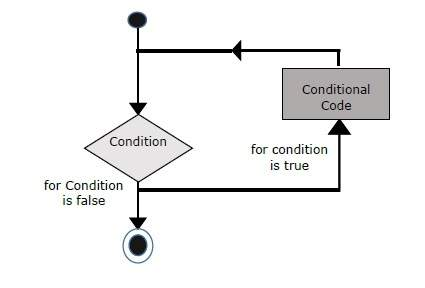# Solidity - For Loop

The for loop is the most compact form of looping. It includes the following three important parts −

• The loop initialization where we initialize our counter to a starting value. The initialization statement is executed before the loop begins.

• The test statement which will test if a given condition is true or not. If the condition is true, then the code given inside the loop will be executed, otherwise the control will come out of the loop.

• The iteration statement where you can increase or decrease your counter.

You can put all the three parts in a single line separated by semicolons.

## Flow Chart

The flow chart of a for loop in Solidity would be as follows −## Syntax

The syntax of for loop is Solidity is as follows −

```for (initialization; test condition; iteration statement) {
Statement(s) to be executed if test condition is true
}
```

## Example

```pragma solidity ^0.5.0;

contract SolidityTest {
uint storedData;
constructor() public{
storedData = 10;
}

function getResult() public view returns(string memory){
uint a = 10;
uint b = 2;
uint result = a + b;
return integerToString(result);
}

function integerToString(uint _i) internal pure
returns (string memory) {
if (_i == 0) {
return "0";
}
uint j=0;
uint len;
for (j = _i; j != 0; j /= 10) {  //for loop example
len++;
}
bytes memory bstr = new bytes(len);
uint k = len - 1;
while (_i != 0) {
bstr[k--] = byte(uint8(48 + _i % 10));
_i /= 10;
}
return string(bstr);//access local variable
}
}
```

Run the above program using steps provided in Solidity First Application chapter.

## Output

```0: string: 12
```
solidity_loops.htm